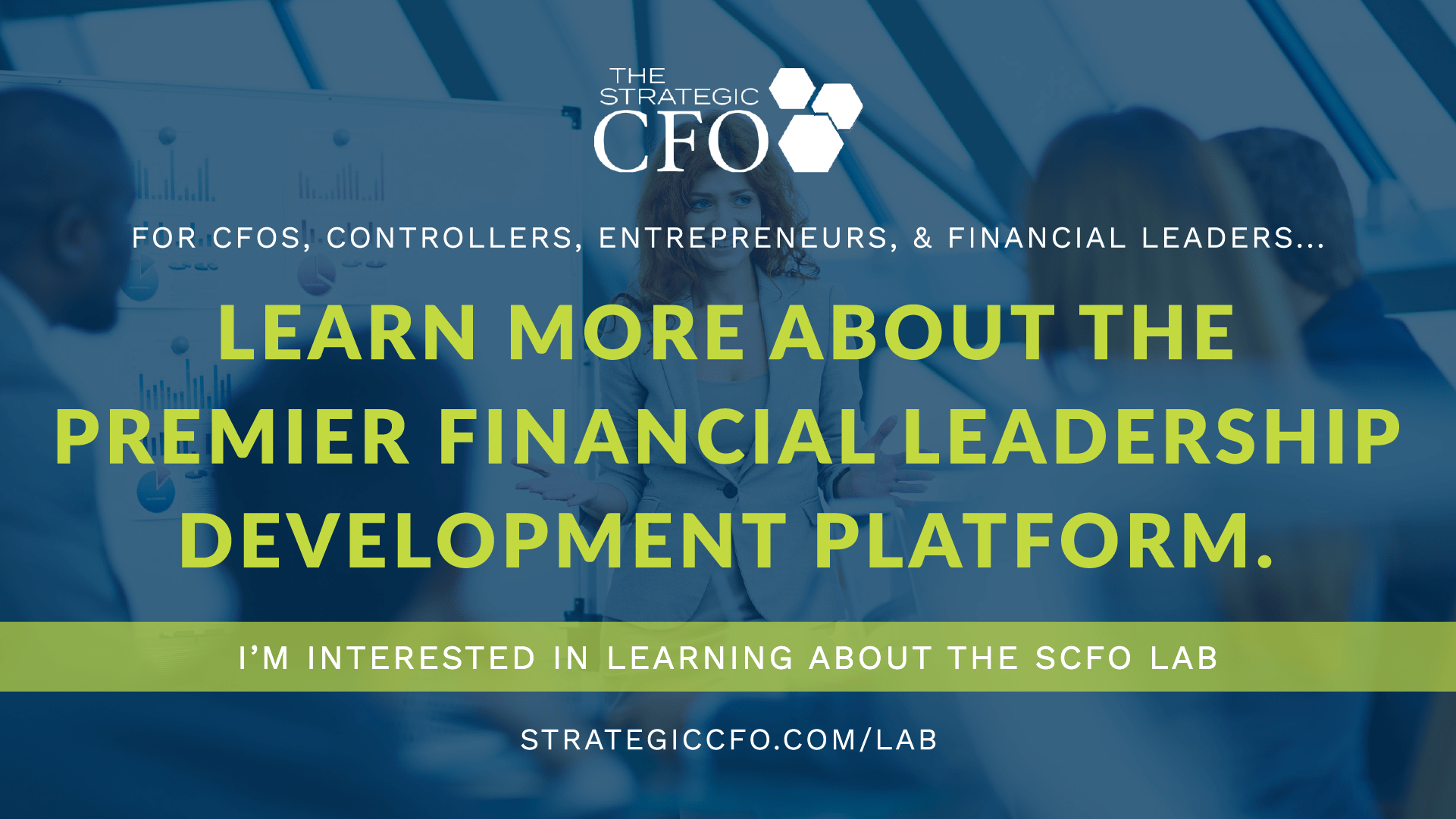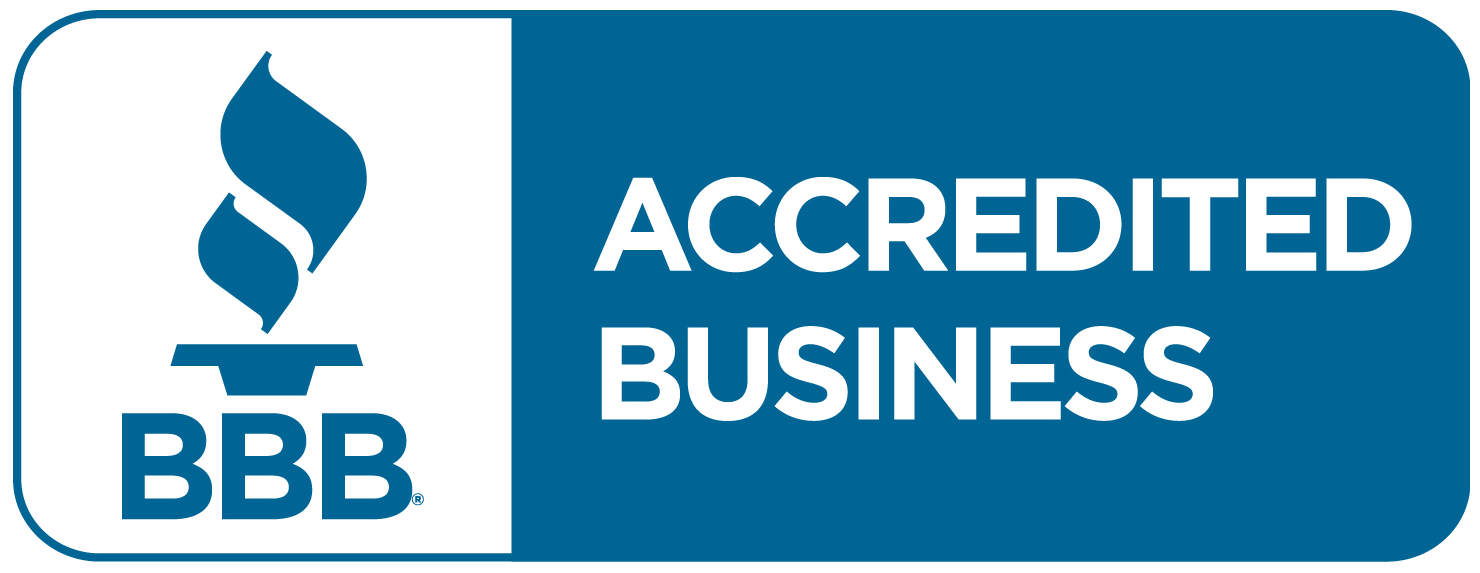What is Compound Interest?

# What is Compound Interest?

Compound interest is interest earned on the principal plus interest earned on prior interest. Compounding interest rates not only earn interest on the original money, but also on the interest itself. The interest earns interest. Or, as Benjamin Franklin put it, “The money that money makes, makes money.”
For example, if an investor invests \$100 at a 10% interest rate compounded yearly, during the first year the investment would earn interest on the original \$100, and the next year the investment would earn interest on the original \$100 plus the \$10 of interest earned in the prior year.

## Compounding Periods

You can compound compound interest at different intervals, such as yearly, semi-annually, quarterly, monthly, daily, or continuously.
For yearly compounding interest rates, the original capital earns interest at the stated annual rate over the course of the year. The following year, the interest earned during the first year is added to the original capital, and the investment earns interest on the new amount.
For semi-annual, quarterly, monthly, or daily compounding interest rates, the original capital earns interest for the stated time period. At the end of that stated time period, the interest earned is added to the capital, and for the next period interest is earned on that new amount. This continues and the amount of money that earns interest gets larger and larger each period.
For a continuously compounding rate, the compounding period is an instant. In this case, compound the interest an infinite number of times during the course of a year.

## Compound Interest and Simple Interest

There is a difference between compound interest and simple interest. An investment with compound interest grows faster than an investment with simple interest. Simple interest is interest earned on the original amount of capital. Each time period, the stated interest rate applies only to the principal amount. With simple interest, the interest itself does not earn interest.
For example, if \$100 is invested at 10% yearly simple interest rate, then the investment earns \$10 of interest each year. Each year, the interest rate applies only to the original \$100 dollars and not to the accumulating interest.
Compound interest, as stated above, earns interest on the principal as well as the interest earned in prior periods. For example, if an investor invests \$100 at a 10% interest rate compounded yearly, during the first year the investment would earn interest on the original \$100, and the next year the investment would earn interest on the original \$100 plus the \$10 of interest earned in the prior year.

## Compound Interest Formula

Here is how you calculate the value of an investment with compound interest after a certain number of years. First, divide the annual interest rate by the number of compounding periods. Then add one to that number. Next raise that value to the product of the number of compounding periods multiplied by the number of years of the investment. Take the value, and multiply it by the principal value. This gives you the ending value of the investment including compounded interest.

Investment Value = Principal x (1 + (Annual Rate/Periods))periods x years

### Compound Interest Monthly Formula

Use the following formula to calculate compound interest on a monthly basis:

Investment Value = Principal x (1 + (Annual Rate/12))12

For example, if you invest \$100 at an annual rate of 6% that compounds monthly, then at the end of one year, the value of the investment would be \$106.17.

\$106.17 = \$100 x (1 + (.06/12))12

### Compound Interest Formula Quarterly

Use the following formula to calculate compound interest on a quarterly basis:

Investment Value = Principal x (1 + (Annual Rate/4))4

For example, if you invest \$100 at an annual rate of 6% that compounds quarterly, then at the end of one year, the value of the investment would be \$106.14.

\$106.14 = \$100 x (1 + (.06/4))4ARTICLES YOU MIGHT LIKE

### The Struggles of Private Company Accounting

Hiring the right accountant  When I meet a business owner operating at a successful \$10 million in revenue, they often mention, “My CPA”… I immediately know that CEO/Entrepreneur is referring to their Tax CPA.  That is because one thing that all Entrepreneurs have in common is that they must file a tax return.  So, from

### IN CRISIS? GET A STRATEGIC CFO! – CEO Blindspots Podcast

Friend of the firm, Birgit Kamps, recently had Strategic CFO President, Dan Corredor, as a guest on her podcast, CEO Blindspots. CEO BLINDSPOTS HOST: Birgit Kamps. She was speaking five languages by the age of 10, and lived in five countries with her Dutch parents prior to becoming an American citizen. Birgit’s professional experience includes starting

### SHRM calls ICHRA the 401K for Group Health Benefits

Fed-up with group health insurance? ICHRA is the new way to offer great health benefits and avoid ACA penalties, SHRM calls it the 401K for group health benefits.  In 2020 the Department of Labor, HHS and IRS changed the rules for employer health benefits. They changed the Affordable Care Act mandates and penalties for every

MARCH 28TH-31ST 2022

JOIN THE NEXT STRATEGIC CFO™ SERIES

The Art of the CFO®

Days
Hours
Min

September 12-15th 2022